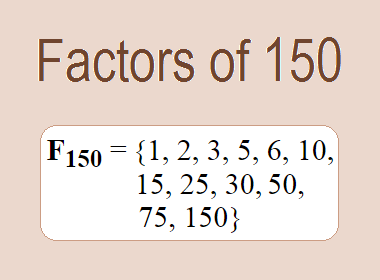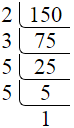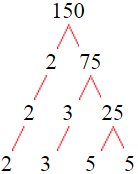# Factors of 150The factors of 150 are 1, 2, 3, 5, 6, 10, 15, 25, 30, 50, 75, and 150 i.e. F150 = {1, 2, 3, 5, 6, 10, 15, 25, 30, 50, 75, 150}. The factors of 150 are all the numbers that can divide 150 without leaving a remainder.

We can check if these numbers are factors of 150 by dividing 150 by each of them. If the result is a whole number, then the number is a factor of 150. Let's do this for each of the numbers listed above:

·        1 is a factor of 150 because 150 divided by 1 is 150.

·        2 is a factor of 150 because 150 divided by 2 is 75.

·        3 is a factor of 150 because 150 divided by 3 is 50.

·        5 is a factor of 150 because 150 divided by 5 is 30.

·        6 is a factor of 150 because 150 divided by 6 is 25.

·        10 is a factor of 150 because 150 divided by 10 is 15.

·        15 is a factor of 150 because 150 divided by 15 is 10.

·        25 is a factor of 150 because 150 divided by 25 is 6.

·        30 is a factor of 150 because 150 divided by 30 is 5.

·        50 is a factor of 150 because 150 divided by 50 is 3.

·        75 is a factor of 150 because 150 divided by 75 is 2.

·        150 is a factor of 150 because 150 divided by 150 is 1.

## How to Find Factors of 150?

1 and the number itself are the factors of every number. So, 1 and 150 are two factors of 150. To find the other factors of 150, we can start by dividing 150 by the numbers between 1 and 150. If we divide 150 by 2, we get a remainder of 0. Therefore, 2 is a factor of 150. If we divide 150 by 3, we get a remainder of 0. Therefore, 3 is also a factor of 150.

Next, we can check if 4 is a factor of 150. If we divide 150 by 4, we get a remainder of 2. Therefore, 4 is not a factor of 150. We can continue this process for all the possible factors of 150.

Through this process, we can find that the factors of 150 are 1, 2, 3, 5, 6, 10, 15, 25, 30, 50, 75, and 150. These are the only numbers that can divide 150 without leaving a remainder.

********************

********************

## Properties of the Factors of 150

The factors of 150 have some interesting properties. One of the properties is that the sum of the factors of 150 is equal to 372. We can see this by adding all the factors of 150 together:

1 + 2 + 3 + 5 + 6 + 10 + 15 + 25 + 30 + 50 + 75 + 150 = 372

Another property of the factors of 150 is that the prime factors of 150 are 2, 3, and 5 only.

## Applications of the Factors of 150

The factors of 150 have several applications in mathematics. One of the applications is in finding the highest common factor (HCF) of two or more numbers. The HCF is the largest factor that two or more numbers have in common. For example, to find the HCF of 150 and 120, we need to find the factors of both numbers and identify the largest factor they have in common. The factors of 150 are 1, 2, 3, 5, 6, 10, 15, 25, 30, 50, 75, and 150. The factors of 120 are 1, 2, 3, 5, 6, 8, 10, 12, 15, 20, 24, 30, 40, 60, and 120. The largest factor that they have in common is 30. Therefore, the HCF of 150 and 120 is 30.

Another application of the factors of 150 is in prime factorization. Prime factorization is the process of expressing a number as the product of its prime factors. The prime factors of 150 are 2, 3, and 5 since these are the only prime numbers that can divide 150 without leaving a remainder. Therefore, we can express 150 as:

150 = 2 × 3 × 5 × 5

We can do prime factorization by division and factor tree method also. Here is the prime factorization of 150 by division method,150 = 2 × 3 × 5 × 5

Here is the prime factorization of 150 by the factor tree method,150 = 2 × 3 × 5 × 5

## Conclusion

The factors of 150 are the numbers that can divide 150 without leaving a remainder. The factors of 150 are 1, 2, 3, 5, 6, 10, 15, 25, 30, 50, 75, and 150. The factors of 150 have some interesting properties, such as having a sum of 372. The factors of 150 have several applications in mathematics, such as finding the highest common factor and prime factorization.